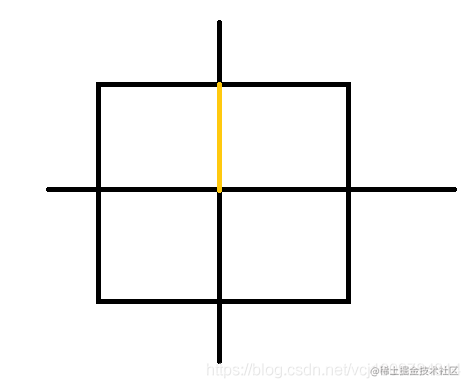算法周赛笔记（7月第4周）— LeetCode 第252场周赛

题目

1952

C++代码

class Solution {
public:
bool isThree(int n) {
for (int i = 2; i <= n / i; i++) {
if (n % i == 0) {
return i == n / i;
}
}
return false;
}
};

1953

• 每周，你将会完成 某一个 项目中的 恰好一个 阶段任务。你每周都 必须 工作。
• 连续的 两周中，你 不能 参与并完成同一个项目中的两个阶段任务。 一旦所有项目中的全部阶段任务都完成，或者仅剩余一个阶段任务都会导致你违反上面的规则，那么你将 停止工作 。注意，由于这些条件的限制，你可能无法完成所有阶段任务。

• 第 1 周，你参与并完成项目 0 中的一个阶段任务。
• 第 2 周，你参与并完成项目 2 中的一个阶段任务。
• 第 3 周，你参与并完成项目 1 中的一个阶段任务。
• 第 4 周，你参与并完成项目 2 中的一个阶段任务。
• 第 5 周，你参与并完成项目 1 中的一个阶段任务。
• 第 6 周，你参与并完成项目 2 中的一个阶段任务。 总周数是 6 。

[0,2,1,2,1,2]

[2,0,1,2,0,1,2,0,1,2,0,2,0,2]

0 0 0 0 0 0 0 0 0

0 x 0 x 0 x 0 x 0 x 0 x 0 x 0 x 0

0 x 0 x 0 x 0 x 0 x 0 x 0

• rest < longest - 1时，答案是 2 * rest + 1

0 1 0 1 0 1 0 1 0 1 0 x 0 x 0 x 0

• rest > longest - 1时，答案也是rest + longest，即全部的球都能被放进答案中。

• rest < longest - 1，此时对rest部分的球，能全部插入，对longest部分的球，能够尽可能地插入（rest + 1），答案是2 * rest + 1
• rest >= longest - 1，此时能够插入全部的球，答案是rest + longest

C++代码如下

class Solution {
public:
long long numberOfWeeks(vector<int>& milestones) {
int n = milestones.size();
int longest = 0; // 最长的
long long rest = 0;
for (int i = 0; i < n; i++) {
rest += milestones[i];
longest = max(longest, milestones[i]);
}
rest -= longest;
if (rest >= longest - 1) return longest + rest;
else return rest * 2 + 1;
}
};

1954

|x|的值定义为：[(r - 1) + r] + [(r - 2) + r] + .. + [1 + r] = r × r + (1 + 2 + ... + r)

C++代码如下：

class Solution {
public:
long long minimumPerimeter(long long neededApples) {
long long sum = 0;
long long i = 0;
while (sum < neededApples) {
++i;
sum += 12 * i * i;
}
return 8 * i;
}
};

1955

• 比方说，[0,1,2] 和 [0,0,1,1,1,2] 是特殊序列。

• 相反，[2,1,0] ， 和 [0,1,2,0] 就不是特殊序列。

- [0,1,2,0,1,2]
- [0,1,2,0,1,2]
- [0,1,2,0,1,2]
- [0,1,2,0,1,2]
- [0,1,2,0,1,2]
- [0,1,2,0,1,2]
- [0,1,2,0,1,2]

• f(i,0)来表示从numsnums[i]中，0型序列的个数；
• f(i,1)来表示从numsnums[i]中，1型序列的个数；
• f(i,2)来表示从numsnums[i]中，2型序列的个数

• nums[i]=0时，可以不使用这个0，则不产生新的0型序列，则f(i,0)至少等于f(i-1,0)；也可以使用这个0，将这个0追加到所有的f(i-1,0)0型序列后面，形成新的0型序列；这个0也可以自己单独成为一个0型序列（不使用i-1之前的任何0型序列）。而对于f(i-1,1)表示的1型序列，这个0没有任何作用，对于f(i-1,2)表示的2型序列，同样没有作用。容易得到此时的状态转移方程为：

f(i,0)=2 * f(i-1,0) + 1

f(i,1)=f(i-1,1)

f(i,2)=f(i-1,2)

• nums[i]=1时，类似的，可以将这个1追加到所有的f(i-1,1)的1型序列后面，形成新的1型序列。而对f(i-1,0)的0型序列，可以追加这个1，形成新的1型序列；对f(i-1,2)的全部2型序列，不产生作用。故此时的状态转移方程为：

f(i,0)=f(i-1,0)

f(i,1)=2 * f(i-1,1) + f(i-1,0)

f(i,2)=f(i-1,2)

• nums[i]=2时，类似的，可以将这个2追加到所有的f(i-1,2)的2型序列后面，形成新的2型序列。而对f(i-1,1)的1型序列，可以追加这个2，形成新的2型序列；对f(i-1,0)，不产生作用。故此时的状态转移方程为:

f(i,0)=f(i-1,0)

f(i,1)=f(i-1,1)

f(i,2)=2 * f(i-1,2) + f(i-1,1)

• nums[i]=0，更新f(i,0)=2 * f(i-1,0) + 1
• nums[i]=1，更新f(i,1)=2 * f(i-1,1) + f(i-1,0)
• nums[i]=2，更新f(i,2)=2 * f(i-1,2) + f(i-1,1)

C++代码如下

class Solution {
public:
int countSpecialSubsequences(vector<int>& nums) {
int MOD = 1e9 + 7;
long long f0 = 0, f1 = 0, f2 = 0;
for(int i = 0; i < nums.size(); i++) {
if(nums[i] == 0) f0 = (2 * f0 + 1) % MOD;
if(nums[i] == 1) f1 = (2 * f1 + f0) % MOD;
if(nums[i] == 2) f2 = (2 * f2 + f1) % MOD;
}
return f2;
}
};

（完）